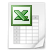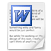### Explanation of the 3x3 magic square

What is a 3x3 magic square?

The 3x3 magic square is square, because it has as many rows (from left to right = horizontal) as columns (from top to bottom = vertical).

The 3x3 magic square consists of 3 rows which multiplied by 3 columns is 9 cells.

The 3x3 magic square must contain 9 different numbers. A pure magic 3x3 square contains the numbers 1, 2, 3, 4, 5, 6, 7, 8 and 9.

The magic square is magic, because the sum of the numbers of each row, each column and both diagonals always give the same result. The sum can be calculated as follows, the (odd) size of the square multiplied by the middle number: 3 x 5 = 15.

What is the secret behind the 3x3 magic square?

The secret behind the 3x3 magic square is easy to explane. If you must take 3 (different) numbers out of the numbers 1 up to 9, which total each time to 15, than there are the following possibilities:

1+5+9

1+6+8

2+4+9

2+5+8

2+6+7

3+4+8

3+5+7

4+5+6

There are 8 possibilities.

The minimum features of the 3x3 square are 3 row features plus 3 column features plus 2 diagonal features total to 8 features. Because there are 8 possibilities and 8 features, there is only one solution of the 3x3 magic square.

If we count the appearance of the 1, 2, 3, 4, 5, 6, 7, 8 and 9 in the 8 possibilities, than we get the following result:

1 =  2x

2 =  3x

3 =  2x

4 =  3x

5 =  4x

6 =  3x

7 =  2x

8 =  3x

9 =  2x

The middle cell takes part of the middle row, the middle column and both diagonals, that is 4 features. That is why you must always put the 5 in the middle cell. The corners take part of one row, one column and one diagonal, that is 3 features. That is why you must put the 2, 4, 6 and 8 (= even numbers) always in the corners. Fill the numbers 1, 3, 7 en 9 in the empty cells (in the middle of the sides). Because you put the 5 in the middle, the sum of the other two cells of a diagonal must be (15 - 5 = ) 10. To get the total of 10 with the even numbers in the corners there are only two possibilities: 2+8 or 4+6. That is why you must put 2 and 8 or 4 and 6 in the same diagonal.

Like I have already told, there is only one solution of the 3x3 magic square, that is excluding rotation and/or mirrorring . Including rotation and/or mirroring (see explanation of the 4x4 magic square) there are (1 x 8 = ) 8 solutions of the 3x3 square.3x3 magic square.xls